# Microwave Hybrid Circuits Notes | Study Basic Electronics Engineering for SSC JE (Technical) - Electrical Engineering (EE)

## Electrical Engineering (EE): Microwave Hybrid Circuits Notes | Study Basic Electronics Engineering for SSC JE (Technical) - Electrical Engineering (EE)

The document Microwave Hybrid Circuits Notes | Study Basic Electronics Engineering for SSC JE (Technical) - Electrical Engineering (EE) is a part of the Electrical Engineering (EE) Course Basic Electronics Engineering for SSC JE (Technical).
All you need of Electrical Engineering (EE) at this link: Electrical Engineering (EE)

Microwave Hybrid Circuits

The interconnection of two or more microwave device using waveguides, tees or direction coupler is called as microwave junction. 

• We use traveling waves as a variables to characterize the hybrid parameters because at microwave range:
(i) It is difficult to mea sure total voltage a nd current at the posrts of network.
(ii) Short and open circuits are difficult to achieve over a broad band of frequencies.

To overcome these problems, we characterize the new parameters called S-parameters.

Waveguide Tees:

E-plane Tee (Series Tee): S13 = – S23 For a matched junction S-matrix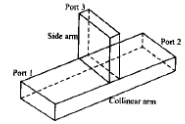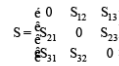S- matrix of E-plane tee is given by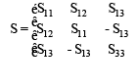H-plane Tee : S13 = S23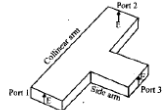S=matrix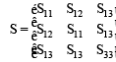Magic Tee (Hybrid Tee) : Combination of E=plane Tee and H-plane Tee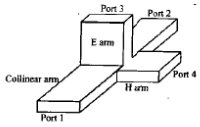1. If input at port 1 and port 2 is applied, the output will be zero at port 3 and additive at port 4.
2. If input is appllied at port 4 it is divided equally between port 1 and port 2 and does not appear at port 3.
3. If input is applied at port 3, it will produce an output of equal magnitude and opposite phase at port 1 and port 2 and output at port 4 is zero i.e. S34 = S43 = 0.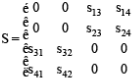Application of Magic Tee: Measurement of impedance, as a mixer, duplexer.
Hybrid rings (Rat-Race circuits):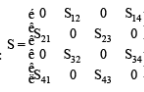S-matrix: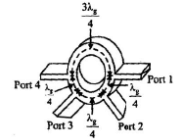If input is at port 1, it will not appear at port 3. If input is at port 2, it will not appear at port 4.

Directional Coupler: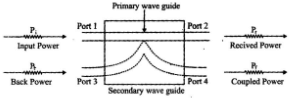Coupling Factor :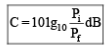Directivity: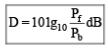Coupling factor is a measure of percentage of incident power being sampled while directivity is a measure of how well the directional coupler distinguish between the forward and the reverse travelling powers.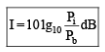Isolation:

S-Matrix: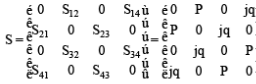Circulator: circulator is a four port microwave device in which wave can only flow from nth port to (n+1)th port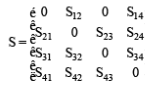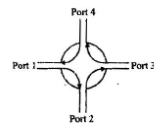Isolator: An isolator is a device which provides very small attenuation in one direction and provides maximum attenuation in the opposite direction. 

• It is uniline device used to improve frequency stability in microwave generators in which the reflection from the load affects the generating frequency.
The document Microwave Hybrid Circuits Notes | Study Basic Electronics Engineering for SSC JE (Technical) - Electrical Engineering (EE) is a part of the Electrical Engineering (EE) Course Basic Electronics Engineering for SSC JE (Technical).
All you need of Electrical Engineering (EE) at this link: Electrical Engineering (EE)Use Code STAYHOME200 and get INR 200 additional OFF

## Basic Electronics Engineering for SSC JE (Technical)

25 docs|22 tests

Track your progress, build streaks, highlight & save important lessons and more!

,

,

,

,

,

,

,

,

,

,

,

,

,

,

,

,

,

,

,

,

,

;